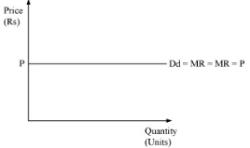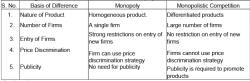Courses

# Test: Non Competitive Markets - 2

## 20 Questions MCQ Test Economics Class 12 | Test: Non Competitive Markets - 2

Description
This mock test of Test: Non Competitive Markets - 2 for UPSC helps you for every UPSC entrance exam. This contains 20 Multiple Choice Questions for UPSC Test: Non Competitive Markets - 2 (mcq) to study with solutions a complete question bank. The solved questions answers in this Test: Non Competitive Markets - 2 quiz give you a good mix of easy questions and tough questions. UPSC students definitely take this Test: Non Competitive Markets - 2 exercise for a better result in the exam. You can find other Test: Non Competitive Markets - 2 extra questions, long questions & short questions for UPSC on EduRev as well by searching above.
QUESTION: 1

Solution:
QUESTION: 2

Solution:
QUESTION: 3

### In perfect competition the goods are

Solution:
QUESTION: 4

Which of the following is the most competitive market structure?

Solution:
QUESTION: 5

In monopolistic competition the goods are

Solution:
QUESTION: 6

A monopoly structure must have one seller, has no substitute, and entry into the industry is preventeD.

Solution:
QUESTION: 7

The market price of the commodity depends on the amount supplied by the monopoly firm.

Solution:

‘Mono’ means one and ‘poly’ means seller. Thus, monopoly refers to a market situation in which there is only one seller of a particular product. Here the firm itself is the industry and the firm’s product has no close substitute. The monopolist is not bothered about the reaction of rival firms since it has none. The demand curve of the monopolist is the industry demand curve. (Recall that in pure competition there are two demand curves).

QUESTION: 8

The market demand curve is the marginal revenue curve for the monopoly firm.

Solution:

In a monopoly market, the marginal revenue curve and the demand curve are distinct and downward-sloping. Production occurs where marginal cost and marginal revenue intersect.

QUESTION: 9

The shape of the total revenue curve depends on the shape of the average revenue curve.

Solution:

Average revenue is the revenue per unit of the commodity sold. It is obtained by dividing the total revenue by the number of units sold. Mathematically AR = TR/Q; where AR = Average revenue, TR = Total revenue and Q = Quantity sold.
If there is any change in the AR, then TR will also change. Therefore, the shape of the total revenue curve depends on the shape of the average revenue curve.

QUESTION: 10

In the case of a negatively sloping straight line demand curve, the total revenue curve is

Solution:
QUESTION: 11

Average revenue for any quantity level can be measured by the slope of the total revenue curve.

Solution:

Average revenue for any quantity level can be measured by the slope of the line from the origin to the relevant point on the total revenue curve.
MR = ∆TR/∆Q
∆TR/∆Q indicates the slope of the total revenue curve.
Thus, if the total revenue curve is given to us, we can find out marginal revenue at various levels of output by measuring the slopes at the corresponding points on the total revenue curve.

QUESTION: 12

Marginal revenue for any quantity level can be measured by the slope of the total revenue curve.

Solution:
QUESTION: 13

Tooth paste industry is an example of?

Solution:

This type of market is combination of monopoly and competitive markets. a monopolistic competitive market is one with freedom of entry and exit, but firms can differentiate their products.
Toothpaste compete on quality of product as much as price. Product differentiation is a key element of the business. There are relatively low barriers to entry in setting up a new business.

QUESTION: 14

Cartels exist in

Solution:

Oligopoly is when a small number of firms collude, either explicitly or tacitly, to restrict output and/or fix prices, in order to achieve above normal market returns. Firms in an oligopoly set prices, whether collectively – in a cartel – or under the leadership of one firm, rather than taking prices from the market.

QUESTION: 15

Under which market conditions firms make only Normal profit in the long run

Solution:
QUESTION: 16

The demand curve of a monopoly firm will be:

Solution:
QUESTION: 17

A firm practicing price discrimination will be?

Solution:
QUESTION: 18

Price discrimination under monopoly depends on?

Solution:

The monopolist has control over pricing, demand, and supply decisions, thus, sets prices in a way, so that maximum profit can be earned. The monopolist often charges different prices from different consumers for the same product. This practice of charging different prices for identical product is called price discrimination. And in monopoly it is decided by the change in the demand of the product.

QUESTION: 19

The AR curve and industry demand curve are same in case of?

Solution:

In a monopoly market, there is only one product or service of such kind, therefore the demand of the product is not affected by any external force which means the AR will remain same as the Demand.

QUESTION: 20

The market structure in which the number of sellers is small and there is interdependence in decision making by the firms is known as

Solution:

Track your progress, build streaks, highlight & save important lessons and more!

### Similar Content### Related tests